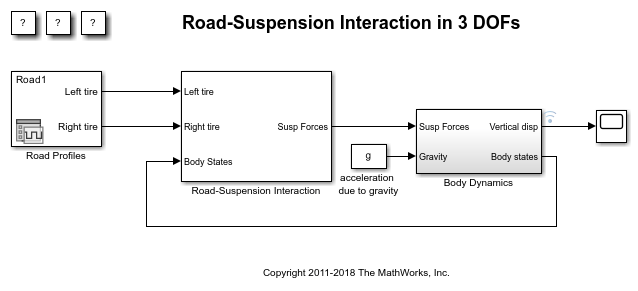# Multiple Simulations Workflow Tips

The goal of this example is to provide helpful tips for running multiple simulations using parallel simulation tools. This example will work even if the Parallel Computing Toolbox™ is not available, but the simulations will run in serial. We will be using the model `sldemo_suspn_3dof`.

```mdl = 'sldemo_suspn_3dof'; isModelOpen = bdIsLoaded(mdl); open_system(mdl); ```### Initializing an Array of Simulink.SimulationInput Objects

Typically you will construct an array of Simulink.SimulationInput objects in order to run multiple simulations. There are several ways to initialize the array before populating it with data.

```numSims = 5; Cf_sweep = Cf*linspace(.05,.95, numSims); ```

Method 1: Initialize the array before the loop

```in(numSims) = Simulink.SimulationInput; for idx = 1:numSims % Need to populate the model name since we get any empty array by default in(idx).ModelName = 'sldemo_suspn_3dof'; in(idx) = in(idx).setVariable('Cf', Cf_sweep(idx)); end ```

Method 2: Initialize the array in the loop

Note that the loop variable `idx` starts from the largest value so that the entire array is pre-allocated.

```for idx = numSims:-1:1 % Since we are indexing from 5 to 1, the first iteration will % initialize the array. in(idx) = Simulink.SimulationInput('sldemo_suspn_3dof'); in(idx) = in(idx).setVariable('Cf', Cf_sweep(idx)); end ```

### Setting Model and Block Parameters

The `setModelParameter` and `setBlockParameter` methods use the same parameter-value pair syntax that the `set_param` API uses. This means that most values you pass in to these methods should be character arrays, not their literal value.

```for idx = numSims:-1:1 in(idx) = Simulink.SimulationInput('sldemo_suspn_3dof'); % Incorrect in(idx) = in(idx).setModelParameter('StartTime', 5); % Correct in(idx) = in(idx).setModelParameter('StartTime', '3'); end ```

### Setting Variables

The `setVariable` method expects that you will pass the literal value you want to assign to a variable. The idea is that this closely reflects the `assignin` syntax.

```for idx = numSims:-1:1 in(idx) = Simulink.SimulationInput('sldemo_suspn_3dof'); % Incorrect, Cf is expected to be a double, not a character array in(idx) = in(idx).setVariable('Cf', '2500'); % Correct, Cf is a scalar double in(idx) = in(idx).setVariable('Cf', 2500); end ```

### Diagnosing Runtime Errors

Assume that you've accidentally set up an array of Simulink.SimulationInput objects with an incorrect value.

```Mb_sweep = linspace(0, 1500, numSims); for idx = numSims:-1:1 in(idx) = Simulink.SimulationInput('sldemo_suspn_3dof'); % Accidentally set the Mass to 0 on the first iteration in(idx) = in(idx).setVariable('Mb', Mb_sweep(idx)); % Shorten the stop time in(idx) = in(idx).setModelParameter('StopTime','1'); end ```

Simulating these will cause a runtime error

```out = sim(in); ```
```[09-Dec-2021 11:45:05] Running simulations... [09-Dec-2021 11:45:26] Completed 1 of 5 simulation runs. Run 1 has errors. [09-Dec-2021 11:45:36] Completed 2 of 5 simulation runs [09-Dec-2021 11:45:36] Completed 3 of 5 simulation runs [09-Dec-2021 11:45:37] Completed 4 of 5 simulation runs [09-Dec-2021 11:45:46] Completed 5 of 5 simulation runs Warning: Simulation(s) with indices listed below completed with errors. Please inspect the corresponding SimulationOutput to get more details about the error:  ```

Fortunately, you can inspect the Simulink.SimulationOutput object to see any error messages that come from a simulation.

```out(1).ErrorMessage ```
```ans = 'Derivative of state '1' in block '<a href="matlab:open_and_hilite_hyperlink ('sldemo_suspn_3dof/Body Dynamics/Vertical (Z) dynamics/Zdot','error')">sldemo_suspn_3dof/Body Dynamics/Vertical (Z) dynamics/Zdot</a>' at time 0.0 is not finite. The simulation will be stopped. There may be a singularity in the solution. If not, try reducing the step size (either by reducing the fixed step size or by tightening the error tolerances)' ```

This works to debug issues on parallel workers too.

```for idx = numSims:-1:1 in(idx) = Simulink.SimulationInput('sldemo_suspn_3dof'); % Accidentally set the Mass to 0 on the first iteration in(idx) = in(idx).setVariable('Mb', Mb_sweep(idx)); % Shorten the stop time in(idx) = in(idx).setModelParameter('StopTime','1'); end out = parsim(in); ```
```[09-Dec-2021 11:45:47] Checking for availability of parallel pool... Starting parallel pool (parpool) using the 'local' profile ... Connected to the parallel pool (number of workers: 6). [09-Dec-2021 11:47:08] Starting Simulink on parallel workers... [09-Dec-2021 11:47:45] Configuring simulation cache folder on parallel workers... [09-Dec-2021 11:47:45] Loading model on parallel workers... [09-Dec-2021 11:48:06] Running simulations... [09-Dec-2021 11:48:18] Completed 1 of 5 simulation runs. Run 1 has errors. [09-Dec-2021 11:48:18] Completed 2 of 5 simulation runs [09-Dec-2021 11:48:18] Completed 3 of 5 simulation runs [09-Dec-2021 11:48:18] Completed 4 of 5 simulation runs [09-Dec-2021 11:48:18] Completed 5 of 5 simulation runs Warning: Simulation(s) with indices listed below completed with errors. Please inspect the corresponding SimulationOutput to get more details about the error:  [09-Dec-2021 11:48:18] Cleaning up parallel workers... ```

Inspecting the Simulink.SimulationOutput reveals a non-finite derivative error.

```out(1).ErrorMessage ```
```ans = 'Derivative of state '1' in block '<a href="matlab:open_and_hilite_hyperlink ('sldemo_suspn_3dof/Body Dynamics/Vertical (Z) dynamics/Zdot','error')">sldemo_suspn_3dof/Body Dynamics/Vertical (Z) dynamics/Zdot</a>' at time 0.0 is not finite. The simulation will be stopped. There may be a singularity in the solution. If not, try reducing the step size (either by reducing the fixed step size or by tightening the error tolerances)' ```

The `applyToModel` method will configure your model with the settings on the SimulationInput so you can debug the problem locally.

```in(1).applyToModel; ```

Notice that the value of the variable `Mb` in the base workspace changes to 0 to reflect the value that was used in the simulation corresponding to the first SimulationInput object in `in`.

### Close MATLAB Workers

Last, close the parallel pool and the model if they were not previously opened.

```if(~isModelOpen) close_system(mdl, 0); end delete(gcp('nocreate')); ```
```Parallel pool using the 'local' profile is shutting down. ```